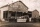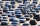# Two cubes

The surfaces of two cubes, one of which has an edge of 22 cm longer than the second are differ by 19272 cm2. Calculate the edge length of both cubes.

Result

a =  84 cm
b =  62 cm

#### Solution:Leave us a comment of example and its solution (i.e. if it is still somewhat unclear...):

Showing 0 comments:Be the first to comment!#### To solve this example are needed these knowledge from mathematics:

Looking for help with calculating roots of a quadratic equation? Do you have a linear equation or system of equations and looking for its solution? Or do you have quadratic equation?

## Next similar examples:

1. PoolIf water flows into the pool by two inlets, fill the whole for 8 hours. The first inlet filled pool 6 hour longer than second. How long pool take to fill with two inlets separately?
2. Two carsTwo cars started against each other at the same time to journey long 293 km. First car went 41 km/h and second 41 km/h. What distance will be between this cars 20 minutes before meet?
3. An investmentHow long will it take for an investment to double at a simple interest rate of 5.10% p. A. ? Express the answer in years and months, rounded up to the next month.
4. Right triangle AlefThe obvod of a right triangle is 84 cm, the hypotenuse is 37 cm long. Determine the lengths of the legs.
5. ObserverThe observer sees straight fence 100 m long in 30° view angle. From one end of the fence is 153 m. How far is it from the another end of the fence?
6. TabithaTabitha manufactures a product that sells very well. The capacity of her facility is 241,000 units per year. The fixed costs are \$122,000 per year and the variable costs are \$11 per unit. The product currently sells for \$17. a. What total revenue is requ
7. Car repairJohn bought a car for a certain sum of money. He spent 10% of the cost to repairs and sold the car for a profit of Rs. 11000. How much did he spend on repairs if he made a profit of 20%?
8. Trapezoid MOThe rectangular trapezoid ABCD with right angle at point B, |AC| = 12, |CD| = 8, diagonals are perpendicular to each other. Calculate the perimeter and area of ​​the trapezoid.
9. BonusGross wage was 527 EUR including 16% bonus. How many EUR were bonuses?
10. LogicA man can drink a barrel of water for 26 days, woman for 48 days. How many days will a barrel last between them?
11. BeerAfter three 10° beers consumed in a short time there are 5.6 g of alcohol in 6 kg adult human blood. How much is it per mille?
12. ClockHow many times a day hands on a clock overlap?
13. ServerCalculate how many average minutes a year is the web server is unavailable, the availability is 99.99%.
14. RiverFrom the observatory 11 m high and 24 m from the river bank, river width appears in the visual angle φ = 13°. Calculate width of the river.
15. Proof PTCan you easy prove Pythagoras theorem using Euclidean theorems? If so, do it.
16. CirclesThe areas of the two circles are in the ratio 2:20. The larger circle has diameter 20. Calculate the radius of the smaller circle.
17. Triangle ABCCalculate the sides of triangle ABC with area 1404 cm2 and if a: b: c = 12:7:18